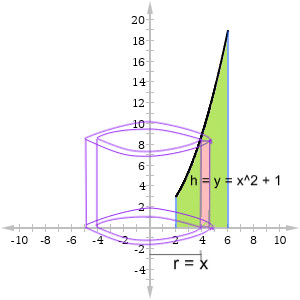$$dV = CS\\ C: perimeter, = 2\pi x\\ S: area, = ydx\\ \Rightarrow dV = 2 \pi x y dx\\$$